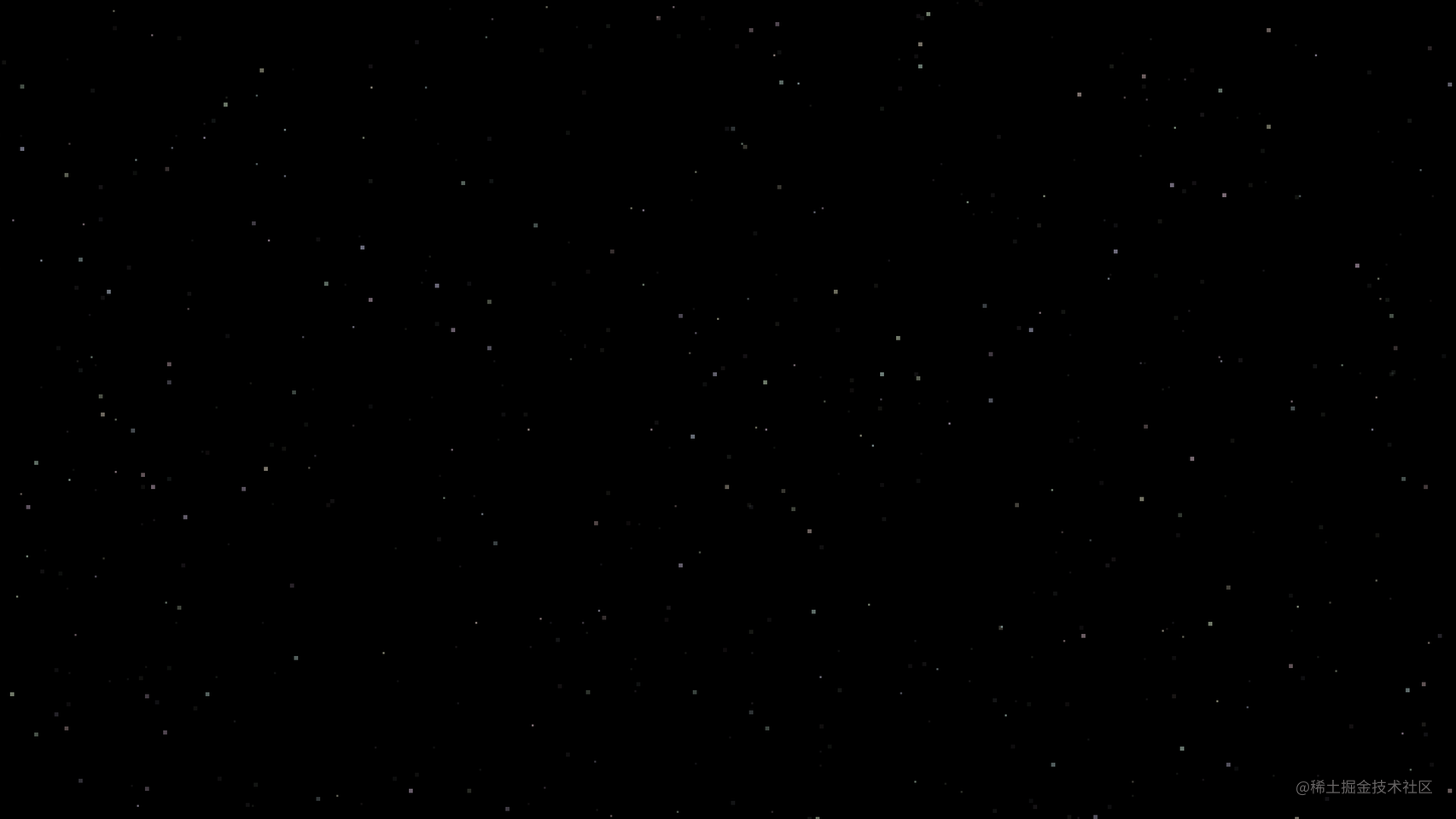# 用CSS Houdini画一片星空

CSS Houdini可以做些什么？谷歌开发者文档列了几个demo，我们先来看一下这几个demo：

（1）给textarea加一个方格背景（demo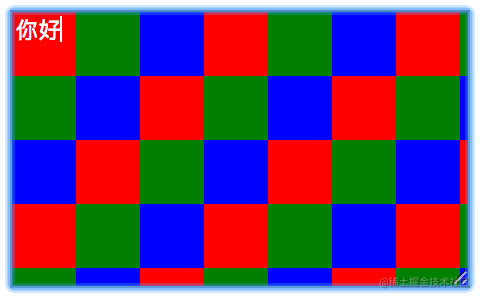``````textarea {
background-image: paint(checkerboard);
}复制代码``````

（2）给div添加一个钻石形状背景（demo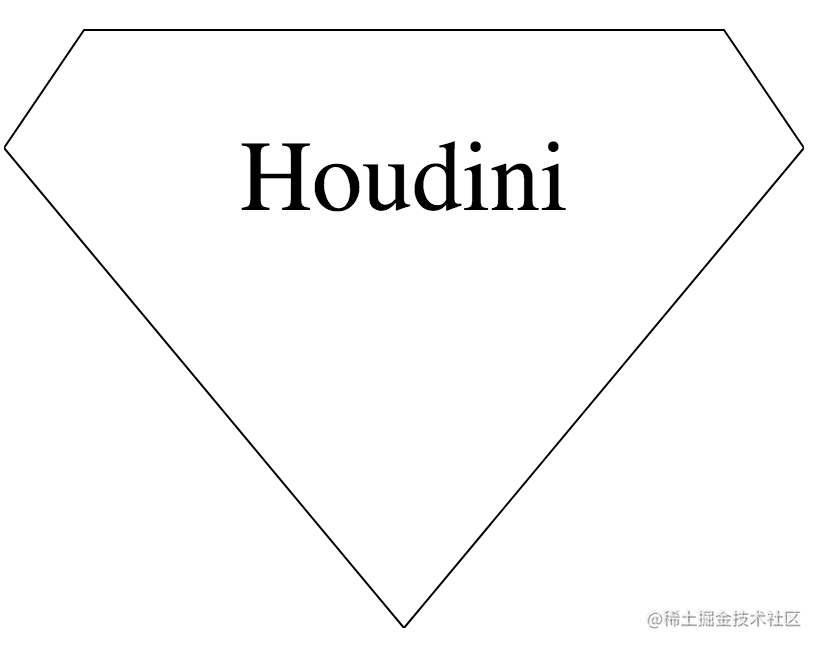``````div {
--top-width: 80;
--top-height: 20;
}复制代码``````

（3）点击圆圈扩散动画（demo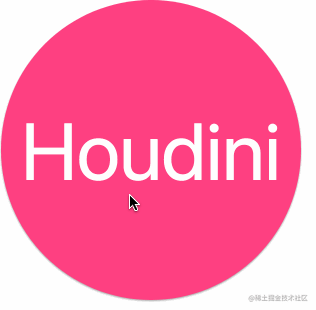（1）需要调好和其它html元素的定位和z-index关系等

（2）编辑框等不能方便地改背景，不能方便地做变量控制

（3）不能方便地进行复用

CSS Houdini在解决这种自定义图形图像绘制的问题提供了很好的解决方案，可以用Canvas画一个你想要的图形，然后注册到CSS系统里面，就能在CSS属性里面使用这个图形了。以画一个星空为例，一步步说明这个过程。

### 1. 画一个黑夜的夜空

CSS Houdini只能工作在localhost域名或者是https的环境，否则的话相关API是不可见（undefined）的。如果没有https环境的话，可以装一个http-server的npm包，然后在本地启动，访问localhost:8080就可以了，新建一个index.html，写入：

``````<!DOCType>
<html>
<meta charset="utf-8">
<style>
body {
background-image: paint(starry-sky);
}
</style>
<body>
<script>
</script>
</body>
</html>复制代码``````

``````body {
background-image: paint(starry-sky);
}复制代码``````

Worklet需要的starry-sky.js的代码如下所示：

``````class StarrySky {
paint (ctx, paintSize, properties) {
// 使用Canvas的API进行绘制
ctx.fillRect(0, 0, paintSize.width, paintSize.height);
}
}
// 注册这个属性
registerPaint('starry-sky', StarrySky);复制代码``````

``````let blobURL = URL.createObjectURL( new Blob([ '(',
function(){

class StarrySky {
paint (ctx, paintSize, properties) {
ctx.fillRect(0, 0, paintSize.width, paintSize.height);
}
}
registerPaint('starry-sky', StarrySky);

}.toString(),

')()' ], { type: 'application/javascript' } )
);

### 2. 画星星

Cavans星星效果网上找一个就好了，例如这个Codepen，代码如下：

``````paint (ctx, paintSize, poperties) {
let xMax= paintSize.width;
let yMax = paintSize.height;

// 黑色夜空
ctx.fillRect(0, 0, xMax, yMax);

// 星星的数量
let hmTimes = xMax + yMax;
for (let i = 0; i <= hmTimes; i++) {
// 星星的xy坐标，随机
let x = Math.floor((Math.random() * xMax) + 1);
let y = Math.floor((Math.random() * yMax) + 1);
// 星星的大小
let size = Math.floor((Math.random() * 2) + 1);
// 星星的亮暗
let opacityOne = Math.floor((Math.random() * 9) + 1);
let opacityTwo = Math.floor((Math.random() * 9) + 1);
let hue = Math.floor((Math.random() * 360) + 1);
ctx.fillStyle = `hsla(\${hue}, 30%, 80%, .\${opacityOne + opacityTwo})`; ctx.fillRect(x, y, size, size); } }复制代码``````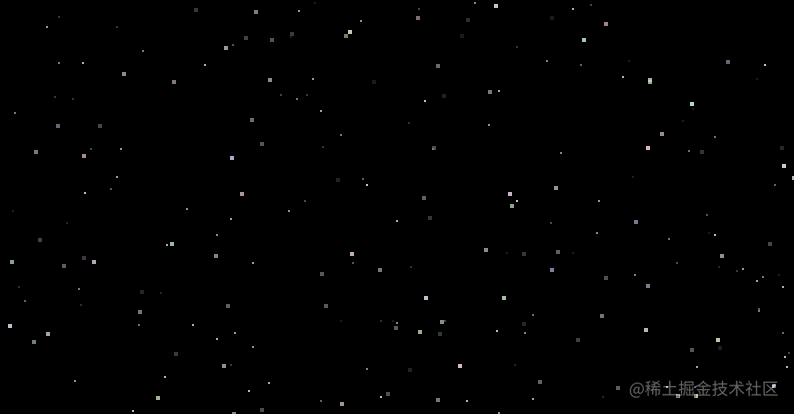### 3. 控制星星的密度

``````body {
--star-density: 0.8;
background-image: paint(starry-sky);
}复制代码``````

``````body:before {
content: "";
position: absolute;
left: 0;
top: 0;
width: 100%;
height: 100%;
--star-density: 0.5;
background-image: paint(starry-sky);
}复制代码``````

``````class StarrySky {
static get inputProperties() {
return ['--star-density'];
}
}复制代码``````

``````class StarrySky {
paint (ctx, paintSize, properties) {
// 获取自定义属性值
let starDensity = +properties.get('--star-density').toString() || 1;
// 最大只能为1
starDensity > 1 && (starDensity = 1);
// 星星的数量剩以这个系数
let hmTimes = Math.round((xMax + yMax) * starDensity);
}
}复制代码``````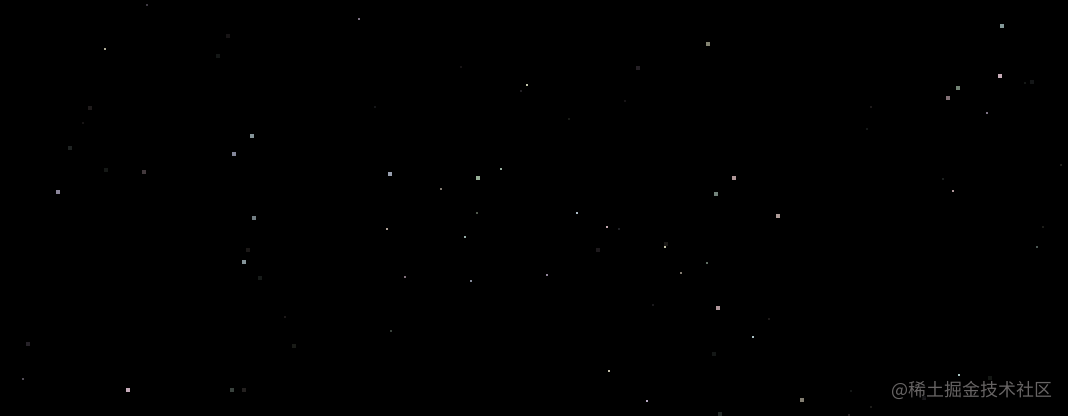### 4. 记录星星的数据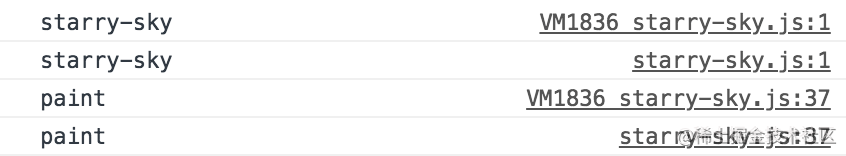``````    random () {
let x = Math.sin(this.seed++) * 10000;
return x - Math.floor(x);
}复制代码``````

``````body:before {
--starry-sky-seed: 1;
--star-density: 0.5;
background-image: paint(starry-sky);
}复制代码``````

``````paint (ctx, paintSize, properties) {
if (!this.stars) {
let starOpacity = +properties.get('--star-opacity').toString();
// 得到随机化种子，可以不传，默认为0
this.seed = +(properties.get('--starry-sky-seed').toString() || 0);
}
}复制代码``````

``````random () {
let x = Math.sin(this.seed++) * 10000;
return x - Math.floor(x);
}

addStars (xMax, yMax, starDensity = 1) {
starDensity > 1 && (starDensity = 1);
// 星星的数量
let hmTimes = Math.round((xMax + yMax) * starDensity);
this.stars = new Array(hmTimes);
for (let i = 0; i < hmTimes; i++) {
this.stars[i] = {
x: Math.floor((this.random() * xMax) + 1),
y: Math.floor((this.random() * yMax) + 1),
size: Math.floor((this.random() * 2) + 1),
// 星星的亮暗
opacityOne: Math.floor((this.random() * 9) + 1),
opacityTwo: Math.floor((this.random() * 9) + 1),
hue: Math.floor((this.random() * 360) + 1)
};
}
}复制代码``````

``````const ONE_HOUR = 36000 * 1000;
this.seed = +(properties.get('--starry-sky-seed').toString() || 0)
+ Date.now() / ONE_HOUR >> 0;复制代码``````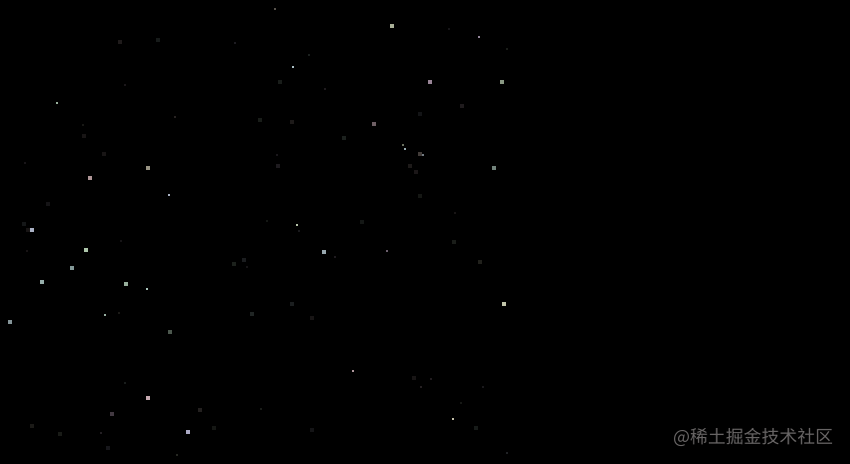### 5. 增量更新星星数据

``````class StarrySky {
constructor () {
// 初始化
this.lastPaintSize = this.paintSize = {
width: 0,
height: 0
};
this.stars = [];
}
}复制代码``````

``````paint (ctx, paintSize, properties) {
// 更新当前paintSize
this.paintSize = paintSize;
// 获取CSS变量设置，把密度、seed等存放到类的实例数据
this.updateControl(properties);
// 增量更新星星
// 黑色夜空
for (let star of this.stars) {
// 画星星，略
}
}复制代码``````

``````updateStars () {
// 如果当前的画布比上一次的要小，则删掉一些星星
if (this.lastPaintSize.width > this.paintSize.width ||
this.lastPaintSize.height > this.paintSize.height) {
this.removeStars();
}
// 如果当前画布变大了，则增加一些星星
if (this.lastPaintSize.width < this.paintSize.width ||
this.lastPaintSize.height < this.paintSize.height) {
}
this.lastPaintSize = this.paintSize;
}复制代码``````

``````removeStars () {
let stars = []
for (let star of stars) {
if (star.x <= this.paintSize.width &&
star.y <= this.paintSize.height) {
stars.push(star);
}
}
this.stars = stars;
}复制代码``````

``````addStars () {
let xMax = this.paintSize.width,
yMax = this.paintSize.height;
// 星星的数量
let hmTimes = Math.round((xMax + yMax) * this.starDensity);
for (let i = 0; i < hmTimes; i++) {
let x = Math.floor((this.random() * xMax) + 1),
y = Math.floor((this.random() * yMax) + 1);
// 如果星星落在上一次的画布内，则跳过
if (x < this.lastPaintSize.width && y < this.lastPaintSize.height) {
continue;
}

this.stars.push({
x: x,
y: y,
size: Math.floor((this.random() * 2) + 1),
// 星星的亮暗
});
}
}复制代码``````

### 6. 让星星闪起来

``````body:before {
--star-opacity: 1;
--star-density: 0.5;
--starry-sky-seed: 1;
background-image: paint(starry-sky);
}复制代码``````

``````// 获取透明度系数
this.starOpacity = +properties.get('--star-opacity').toString();
for (let star of this.stars) {
// 每个星星的透明度都乘以这个系数
let opacity = +('.' + (star.opacityOne + star.opacityTwo)) * this.starOpacity;
ctx.fillStyle = `hsla(\${star.hue}, 30%, 80%, \${opacity})`;
ctx.fillRect(star.x, star.y, star.size, star.size);
}复制代码``````

``````let start = Date.now();
// before无法获取，所以需要改成正常元素
let node = document.querySelector('.starry-sky');
window.requestAnimationFrame(function changeOpacity () {
let now = Date.now();
// 每隔一1s，透明度从0.5变到1
node.style.setProperty('--star-opacity', (now - start) % 1000 / 2 + 0.5);
window.requestAnimationFrame(changeOpacity);
});复制代码``````

``````body:before {
--star-opacity: 1;
--star-density: 0.5;
--starry-sky-seed: 1;
background-image: paint(starry-sky);
animation: shine 1s linear alternate infinite;
}

@keyframes shine {
from {
--star-opacity: 1;
}
to {
--star-opacity: 0.6;
}
}复制代码``````

``````// 10 px
let length = CSS.px(10);
// 在循环里面改length的值，不用自己去拼字符串

``````CSS.registerProperty({
name: '--star-opacity',
// 指明它是一个数字类型
syntax: '<number>',
inherits: false,
initialValue: 1
});复制代码``````

``````body {
background-color: #000;
}
body:before {
background-image: paint(starry-sky);
animation: shine 1s linear alternate infinite;
}

@keyframes shine {
from {
opacity: 1;
}
to {
opacity: 0.6;
}
}复制代码``````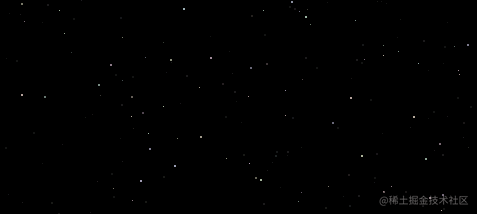【再一次强推书】高效前端已上市，京东、亚马逊、淘宝等均有售